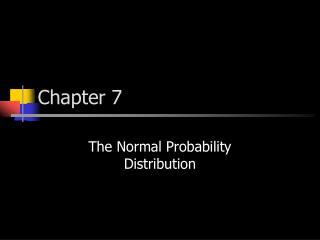DownloadDownload PresentationChapter 7

# Chapter 7

Télécharger la présentation## Chapter 7

- - - - - - - - - - - - - - - - - - - - - - - - - - - E N D - - - - - - - - - - - - - - - - - - - - - - - - - - -
##### Presentation Transcript

1. Chapter 7 The Normal Probability Distribution

2. Review • Continuous Random Variables • Normal Distribution • z values

3. Continuous Distributions • The area under the curve, above an interval on the number line, is the probability of observing a value in that interval. • AREA = PROBABILITY

4. The Standard Normal Table • The Standard Normal Table on the front cover of your book gives the area between z = 0 (the mean) and the z value you provide. • Since normal curves are symmetric, there is no need for the table to list negative z values. You, however, must be very careful to consider the sign of your z values.

5. DON’T MEMORIZE!!! Every semester, students try to memorize a system for determining area. This leads to almost certain doom, as no such system exists. Area is given by the chart – period. You MUST read each problem carefully to determine what area you should look up, and what you should do when you’ve found it.

6. Normal Distributions • Normal distributions come in all shapes and sizes. Fortunately, they can all be transformed into the same distribution – the standard normal distribution – by using z values.

7. Normal Approximation to the Binomial Distribution • Another neat trick involving normal curves is that they can be used to approximate binomial distributions. • Remember, however, that you must adjust for the fact that you are using a continuous distribution to approximate a discrete one.

8. Normal Approximation to the Distribution of Proportions • Proportions are closely linked to binomial distributions. Accordingly, normal curves can be used to approximate the distribution of proportions.

9. The Normal Curve • Faster than a speeding bullet! • More powerful than a locomotive! • Able to leap tall buildings in a single bound! • It slices! • It dices! • It juliennes fries! • It can set the clock on your VCR!

10. Try it! • Find the area under the normal curve between z = 1.20 and z = 2.31. • .4896 - .3849 = .1047

11. Try it! • Find the area under the normal curve to the left of z = -.25. • .5 - .0987 = .4013

12. Try it! • The area between ±z is .9800. What is z? • 2.33

13. Try it! • A normal distribution has a mean of 12.8 and a standard deviation of 2.2. Find the probability that a value chosen at random from this distribution is between 12.8 and 14.8. • .3186

14. Try it! • It has been estimated that only 15% of the administrators in education are “very well qualified”. An educational conference drew 40 administrators. What is the probability that 10 or more are “very well qualified”? • .0606

15. Try it! • Thirty five percent of all statistics students at Grossmont College also attend San Diego State University. One hundred seventy five Grossmont students are randomly selected, what is the probability that less than 40% attend San Diego State? • .9177